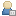###Show Posts

This section allows you to view all posts made by this member. Note that you can only see posts made in areas you currently have access to.

### Messages - Joseph Hussain

Pages: 
1
##### w3DNA -- web interface to 3DNA / Re: DNA bend angle calculation
« on: May 11, 2013, 06:26:17 am »
Hi,

the vectors we used were

HETATM 9998  XS    X X 999      68.227   2.641 -26.513
HETATM 9999  XE    X X 999      67.797  -2.012  34.748

and the output obtained from w3dna is followed

Global linear helical axis defined by equivalent C1' and RN9/YN1 atom pairs
Deviation from regular linear helix: 3.42(0.40)
Helix:    -0.007  -0.076   0.997
HETATM 9998  XS    X X 999      68.227   2.641 -26.513
HETATM 9999  XE    X X 999      67.797  -2.012  34.748
Average and standard deviation of helix radius:
P: 9.51(0.62), O4': 6.66(0.67),  C1': 6.05(0.65)Helix:    -0.007  -0.076   0.997

we have normalized the vectors and got the dot product of two vectors and applied acos on the result of dot product of two vectors and then multiplied with 180/Pi. Precisely the following equation we have used to calculate the bend angle

acos(dot(a, b)) * 180/pi

Thank you

Joseph Hussain

2
##### w3DNA -- web interface to 3DNA / DNA bend angle calculation
« on: May 10, 2013, 12:03:18 am »
We are trying to calculate DNA bend angle using the output obtained from
X3DNA online software, we use the equation given below to solve the
problem and we were unsuccessful.

acos(dot(a, b)) * 180/pi      as mentioned in the blog of Prof. Xiang-Jan Lu

Global linear helical axis defined by equivalent C1' and RN9/YN1 atom pairs
Deviation from regular linear helix: 3.42(0.40)
Helix:    -0.007  -0.076   0.997
HETATM 9998  XS    X X 999      68.227   2.641 -26.513
HETATM 9999  XE    X X 999      67.797  -2.012  34.748
Average and standard deviation of helix radius:
P: 9.51(0.62), O4': 6.66(0.67),  C1': 6.05(0.65)Helix:    -0.007  -0.076   0.997